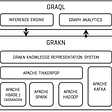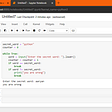# An introduction to linear algebra using Python

Numerous problems in engineering and science can be described or approximated by linear relationships. For example, in finance,physics,biology and other form of sciences. In fact, it is difficult to think of any science or engineering field in which relationships of these kind are not fundamental.

The study of linear relationship is contained in the field of linear algebra, and this article provides a basic overview of some fundamental linear algebraic concepts that are important for scientific computations. Since this text assumes that reader has a prior knowledge of linear algebra, some of the more abstract mathematical concepts and proofs on this topic have been omitted to make the material more accessible,hence we focus on programming linear algebraic computations using python.

You should know Python’s functions for these concepts and calculations.we will be using numpy library and you should know what systems of linear equations are and their relationship to matrices and linear transformations. Finally, you should know how to use Python to compute solutions to systems of linear equations.

# Basics of Linear Algebra

A vector in R is an n-tuple, or point, in R. Vectors can be written horizontally (i.e., with the elements of the vector next to each other) in a row vector, or vertically (i.e., with the elements of the vector on top of each other) in a column vector.

The transpose of a column vector is a row vector of the same length, and the transpose of a row vector is a column vector.

Now we try to create a row vector and column vector, and show the shape of the vectors.

`import numpy as npvector_row = np.array([[1, -5, 3, 2, 4]])vector_column = np.array([,                           ,                           ,                           ])print(vector_row.shape)print(vector_column.shape)`

The result will be :

`(1, 5)(4, 1)`

The transpose of a column vector is a row vector of the same length, and the transpose of a row vector is a column vector.

The norm of a vector is a measure of its length. There are many ways of defining the length of a vector depending on the metric used (i.e., the distance formula chosen). The most common is called the L2 norm.

The L2 norm of a vector v is denoted by:

In general, the p-norm, Lp, of a vector is :

Now we try to transpose the row vector we defined above into a column vector and calculate the L1, L2.

`from numpy.linalg import normnew_vector = vector_row.Tprint(new_vector)norm_1 = norm(new_vector, 1)norm_2 = norm(new_vector, 2)print('L_1 is: %.1f'%norm_1)print('L_2 is: %.1f'%norm_2)`

The result will be :

`[[ 1] [-5] [ 3] [ 2] [ 4]]L_1 is: 15.0L_2 is: 7.4`

The dot product of two vectors is the sum of the product of the respective elements in each vector and is denoted by :

The angle between two vectors, θ, is defined by the formula:

Now we try to compute the angle between the vectors v=[10,9,3] and w=[2,5,12].

`from numpy import arccos, dotv = np.array([[10, 9, 3]])w = np.array([[2, 5, 12]])theta = arccos(dot(v, w.T)/(norm(v)*norm(w)))print(theta)`

The result will be :

`[[0.97992471]]`

Finally, the cross product between two vectors, v and w is defined by :

where θ is the angle between the v and w (which can be computed from the dot product) .

Now given the vectors v=[0,2,0]v=[0,2,0] and w=[3,0,0]w=[3,0,0],we use the Numpy function cross to compute the cross product of v and w.

`v = np.array([[0, 2, 0]])w = np.array([[3, 0, 0]])print(np.cross(v, w))`

The result will be :

`[[ 0  0 -6]]`

# Matrices

An m×n matrix is a rectangular table of numbers consisting of m rows and n columns. The norm of a matrix can be considered as a particular kind of vector norm, if we treat the m×n elements of M are the elements of an mn dimensional vector, then the p-norm of this vector can be write as:

Matrix addition and scalar multiplication for matrices work the same way as for vectors. However, matrix multiplication between two matrices, P and Q, is defined when P is an m×p matrix and Q is a p×n matrix. The result of M=PQ is a matrix M that is m×n which is formally defined as below:

Let the Python matrices P=[[1,7],[2,3],[5,0]] and Q=[[2,6,3,1],[1,2,3,4]].Now we compute the matrix product of P and Q.

`P = np.array([[1, 7], [2, 3], [5, 0]])Q = np.array([[2, 6, 3, 1], [1, 2, 3, 4]])print(P)print(Q)print(np.dot(P, Q))`

The result will be :

`[[1 7] [2 3] [5 0]][[2 6 3 1] [1 2 3 4]][[ 9 20 24 29] [ 7 18 15 14] [10 30 15  5]]`

Using Python to find the determinant of the matrix M=[[0,2,1,3],[3,2,8,1],[1,0,0,3],[0,3,2,1]]. Use the np.eye function to produce a 4×4 identity matrix, I. Multiply M by I to show that the result is M.

`from numpy.linalg import detM = np.array([[0,2,1,3],              [3,2,8,1],              [1,0,0,3],             [0,3,2,1]])print('M:\n', M)print('Determinant: %.1f'%det(M))I = np.eye(4)print('I:\n', I)print('M*I:\n', np.dot(M, I))`

The result will be :

`M: [[0 2 1 3] [3 2 8 1] [1 0 0 3] [0 3 2 1]]Determinant: -38.0I: [[1. 0. 0. 0.] [0. 1. 0. 0.] [0. 0. 1. 0.] [0. 0. 0. 1.]]M*I: [[0. 2. 1. 3.] [3. 2. 8. 1.] [1. 0. 0. 3.] [0. 3. 2. 1.]]`

The inverse of a square matrix M is a matrix of the same size, N, such that M⋅N=I.

Now we try to inverse given matrix below :

`from numpy.linalg import invM = np.array([[0,2,1,3],              [3,2,8,1],              [1,0,0,3],             [0,3,2,1]])print('Inv M:\n', inv(M))`

The result will be :

`Inv M: [[-1.57894737 -0.07894737  1.23684211  1.10526316] [-0.63157895 -0.13157895  0.39473684  0.84210526] [ 0.68421053  0.18421053 -0.55263158 -0.57894737] [ 0.52631579  0.02631579 -0.07894737 -0.36842105]]`

The rank of an m×n matrix A is the number of linearly independent columns or rows of A, and is denoted by rank(A). It can be shown that the number of linearly independent rows is always equal to the number of linearly independent columns for any matrix.

Given matrix A=[[1,1,0],[0,1,0],[1,0,1]], we try to compute the rank for this matrix.

`from numpy.linalg import matrix_rankA = np.array([[1,1,0],              [0,1,0],              [1,0,1]])print('Rank:\n', matrix_rank(A))`

The result will be :

`Rank: 3`

We wish to escalate public knowledge about computer science topics.NetCoinCapital is a blockchain start up which is motivated to expand the bounds of science by trending,researching and developing state of art technologies for future concepts.

--

--

--

## More from Netcoincapital Official

Does small or a big role matter? Anyone who puts all his energy into his position will benefit by reaching the goal. https://linktr.ee/socialmediasNCC

Love podcasts or audiobooks? Learn on the go with our new app.

## Mobcrush Studio is NEW and IMPROVED!## Get Started with GRAKN.AI## Integrating Plaid into a Serverless React App## Pragmatic iOS app architecture## Where to learn programming Or Get New Skills?## Tensorflow is Sponsoring a Kaggle Competition## Web-Socket Overview## How to Prevent Your Integrations From Failing Silently## Netcoincapital Official

Does small or a big role matter? Anyone who puts all his energy into his position will benefit by reaching the goal. https://linktr.ee/socialmediasNCC

## Parametric VS Non-Parametric Methods## Drawings with Python Turtle## Python Template & Concept of Do while loop in Python.